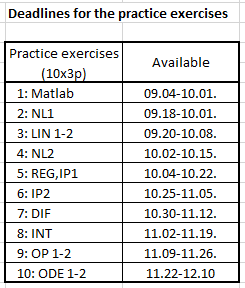# General Information### First steps

- Intsall Matlab to your computer using a BME email address or use online Matlab (see below) (you will need the Symbolic Math, Optimization, Global optimization, Mapping, Image Processing, Curve Fitting, Statistics and Machine Learning Toolboxes)

- Please  create a MathWorks Account and solve the Matlab Onramp online tasks! (see below at Practice exercises)

- Check the Detailed course schedule

- Read and solve tasks from the subject's web page if you missed some classes or check the folder of your lecturer for more actual material.

- If you missed a lesson watch the videos below (Recorded lectures from 2020/21.)# Practice exercises

To ensure the continuous progress from numerical methods subject 30% of the subject points can be obtained by solving small, Matlab practice exercises at home. These exercises are short tasks related to a specific topic. 3-3 points can be achieved by solving the tasks and they are available for at least 1 week after the related topic. Each task can be solved twice, the last result counts. The first task is a bit longer, this is the Matlab Onramp solution for practicing the basics of Matlab. It takes a total of 2 hours, for which 4 weeks are available from the beginning of the semester.# Midterm samples